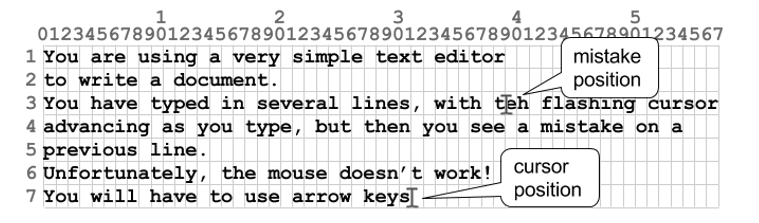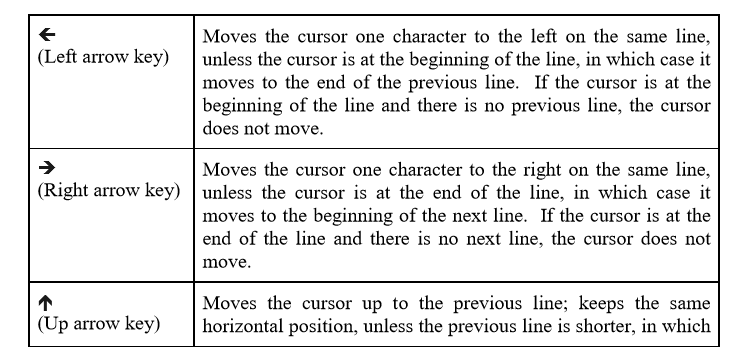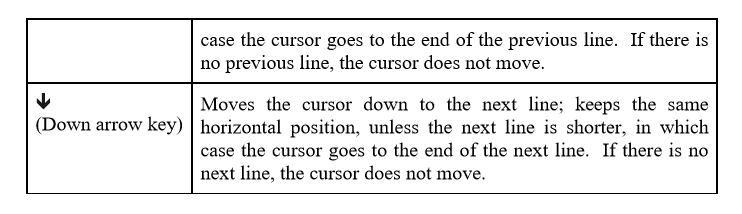## 题目链接：https://nanti.jisuanke.com/t/44820

You are using a very simple text editor to create a document. You have typed in several lines, with the flashing cursor advancing as you type, but then you see a mistake on a previous line. Unfortunately, the mouse doesn’t work! You will have to press arrow keys to move the cursor back to the position where you can fix the mistake. Of course, you want to get to this position as quickly as possible.This simple editor uses a monospace font, so each character is exactly the same width. The cursor can be at the beginning of the line (before the first character), end of the line (after the last character), or at a horizontal position between two characters on a given line. The following keys can be pressed to move the cursor (each keypress is independent of any preceding keypress):The Problem:

Given the line lengths of a text file that was loaded in this simple editor, along with the current cursor position and a different, desired cursor position (e.g., to fix a mistake), you are to determine the minimum number of keypresses, using arrow keys only, required to move the cursor to the desired position.

The Input:

The first input line contains a positive integer, n, indicating the number of editor navigation scenarios to process. Each scenario will occupy exactly 4 input lines. The first line of each scenario contains an integer f (1 ≤ f ≤ 120), indicating the number of lines of text in the file that is loaded in the editor. The next input line contains f integers, s1 to sf, where each value si (0 ≤ si ≤ 80) indicates the number of characters on line i of the file; the values will be separated by exactly one space. A value si = 0 means that there are no characters on line i. The newline character (common character indicating end of a line) does not count as a character on the line. The third input line of each scenario will contain two integers (separated by a space) providing the current cursor position in the file: rc (1 ≤ rc ≤ f) and cc (0 ≤ cc ≤ 80), where rc represents the line of the file, counting from 1 (as with i), and cc represents the horizontal position of the cursor on that line, 0 for the beginning (before the first character). It is guaranteed that the cursor position is valid, so if, for instance, rc = 17 and s17 = 56, then 0 ≤ cc ≤ 56; the maximum value of cc is the end of the line. The fourth input line of each scenario is similar to the third and indicates the desired cursor position to begin fixing the mistake, i.e., this line consists of two integers separated by a space, rm (1 ≤ rm ≤ f) and cm (0 ≤ cm ≤ 80). The constraints on the values for rc and cc also apply to rm and cm.

The Output:

For each scenario in the input, output a single integer on a line by itself indicating the minimum number of keypresses needed to move the cursor from its current position (rc, cc) to the desired position (rm, cm) for fixing the mistake.

2
7
39 20 57 54 14 38 31
7 31
3 39
3
15 30 20
1 12
3 3

21
8

For Case #1, one possible sequence for the minimum number of keypresses to move the cursor from its current position to the desired position is: Up, Up, Right, Up, Left, Up, Left 15 times.

## 收获（教训）

1. 两个if代码块中均存在的变量名不要重复使用，因为可能会把变量修改了，对下一个if语句的判断会有影响（debug的时候发现一次BFS似乎连续走了两个点）
2. 还是要注意读题，这题与普通BFS不同的地方就在于上下移动时可能改变列下标，左右移动时也可能改变行下标（在一行的最左边再左移，将跳到上一行的末尾）。
3. 注意方向，x-1是向上走（注意这个图的坐标不是按平面直角坐标系画的）
4. 当起点在下方，终点在上方时，垂直方向不应该限定只能往上走，因为这题在垂直方向走可能把列下标改变很多，从而得到最优解。

## AC代码

#include <bits/stdc++.h>
using namespace std;
const int N=125,M=85;
int T,n,bx,by,ex,ey,num[N];
bool vis[N][M];
int dir={-1,0,1,0,0,-1,0,1};//上、下、左、右(注意向上走是x-1)
struct node
{
int x,y,cnt;
};
void bfs()
{
queue<node>q;
q.push({bx,by,0});
memset(vis,0,sizeof(vis));
vis[bx][by]=1;
while(!q.empty())
{
node tmp=q.front();q.pop();
int x=tmp.x;
int y=tmp.y;
int cnt=tmp.cnt;
//printf("x=%d y=%d cnt=%d\n",x,y,cnt);
if(x==ex&&y==ey){printf("%d\n",cnt);return;}
for(int i=0;i<4;i++)
{
int nx=x+dir[i];
int ny=y+dir[i];
if(nx>=1&&nx<=n)
{
if(i==0||i==1)//上下移动
{
if(ny<=num[nx]&&!vis[nx][ny])//在长度范围内，直走
{
vis[nx][ny]=1;
q.push({nx,ny,cnt+1});
}
if(ny>num[nx]&&!vis[nx][num[nx]])//长->短
{
vis[nx][num[nx]]=1;
q.push({nx,num[nx],cnt+1});
}
}
else//左右移动 i=2左移 i=3右移
{
if(ny==-1&&i==2&&x-1>=1)//左移到开头前一个，则跳转到上层最后一个
{
int tx=x-1;//注意一定要换个变量，别用nx了，否则后面可能还会改变这个变量
int ty=num[tx];
if(!vis[tx][ty])
{
vis[tx][ty]=1;
q.push({tx,ty,cnt+1});
}
}
if(ny==num[x]+1&&i==3&&x+1<=n)//右移到行尾后一个，则跳转到下层第一个
{
int tx=x+1;
int ty=0;
if(!vis[tx][ty])
{
vis[tx][ty]=1;
q.push({tx,ty,cnt+1});
}
}
if(ny>=0&&ny<=num[x]&&!vis[x][ny])//本行内左右移动
{
vis[x][ny]=1;
q.push({x,ny,cnt+1});
}
}
}
}
}
}
int main()
{
ios::sync_with_stdio(false);
cin>>T;
while(T--)
{
cin>>n;
for(int i=1;i<=n;i++)
cin>>num[i];
cin>>bx>>by>>ex>>ey;
bfs();
}
return 0;
}
/*
1
3
15 30 20
1 12
3 3
ans:8

1
2
1 1
1 0
2 1
ans:2

1
4
1 2 3 4
1 0
4 3
ans:5
*/